from fastai.text.all import *
from torch.utils.data import Dataset
from reformer_fastai.transformer import TransformerLM
from reformer_fastai.reformer import LSHLM## Create dataset

We want to create sequences of the form 0w0w, where w is a sequence of integeres between 1-127 of some lenght: eg. 08470847. We create items on the fly instead of all items up front. We return a tuple to make the dataloader a bit easier to inspect.

class TwinSequence(Dataset):
def __init__(self, sl=1024, len=100):
assert sl%2 == 0
self.sl = sl
self.len = len
def __getitem__(self, idx):
seq = torch.randint(1,128,(self.sl//2,))             # w: [1-127] of len sl//2
seq = 0                                           # seq = 0w
seq = torch.cat((seq,seq), -1)                       # seq = 0w0w
target = torch.cat((seq[1:],torch.tensor()), -1)  # return offset target x:, y:
return (seq, target)
def __len__(self):
return self.len

dls = DataLoaders.from_dsets(TwinSequence(10, 50), bs=6, shuffle=False, device='cuda')
xb, yb = dls.one_batch()
xb.shape, yb.shape

(torch.Size([6, 10]), torch.Size([6, 10]))

Note that the final target item is a padded 0. But that should also be predicitable from the first part of the input sequence:

xb.tolist(), yb.tolist()

([0, 56, 58, 55, 119, 0, 56, 58, 55, 119],
[56, 58, 55, 119, 0, 56, 58, 55, 119, 0])

The number of batches in train data loader (n_iter in fastai lingo): The reformer paper mentions 150 k steps. One step is one iteration/batches.

len(dls.train)

8

We have to mask the first half of the targets. The first part is just random integers, so it's impossible to learn anything from it. We set the tokens in the first part to a special index, -100, and later tell our lossfunction to ignore items with this value. This means that the only task the model can learn is to copy the first part of the input sequence. If we didn't mask the first part, it would be penalized for poor performance in the first part, and would try to find a compromise.

class MaskTargCallback(Callback):
def before_batch(self):
self.y[:, :self.dls.train_ds.sl//2] = -100


We create a custom accuracy that also disregards tokens with value -100:

def masked_accuracy(inp, targ, ignore=-100):
pred = inp.argmax(dim=-1)

pred = torch.tensor([   0,   1,   2,   3,   4,1,2,3,4,55])[None,:]
targ = torch.tensor([-100,-100,-100,-100,-100,1,2,3,4,0])[None,:]

tensor(0.8000)

And finally a callback to inspect items directly before modelling:

class Inspect_items(Callback):
def after_batch(self):
if self.iter==0 and self.epoch==0 and self.training:
inp = self.learn.x.tolist()
targ = self.learn.y.tolist()
df = pd.DataFrame((inp,targ)).T
df.columns = ['inp', 'targ']
print(df)


Let's check what's actually going into the model with a tiny example:

bs, sl = 64,16
n_epochs = 1
train_sz = 500
valid_sz = 100

dls = DataLoaders.from_dsets(TwinSequence(sl, train_sz), TwinSequence(sl, valid_sz), bs=bs, shuffle=False, device='cuda')

model = TransformerLM(128, 256, d_ff=256, n_layers=1, n_heads=4, max_seq_len=sl, pos_enc='fixed',
attn_dropout=0, ff_dropout=0, emb_dropout=0)

learn = Learner(dls, model, loss_func=CrossEntropyLossFlat(ignore_index=-100),

learn.fit(1, 1e-3)

0 4.671275 4.565497 0.125000 00:02
    inp  targ
0     0  -100
1    99  -100
2    69  -100
3    65  -100
4     8  -100
5    51  -100
6     6  -100
7    83  -100
8     0    99
9    99    69
10   69    65
11   65     8
12    8    51
13   51     6
14    6    83
15   83     0


Looks good!

## Short sequence modelling

First we will test a short sequence to test that everything is working. We use the TransformerLM and compares it to the LSHLM. Note that LSHLM has the same LSHAttention as the ReformerLM, but less the other reformer memory tricks.

bs, sl = 64,64
n_epochs = 1
train_sz = 50_000
valid_sz = 10_000
dls = DataLoaders.from_dsets(TwinSequence(sl, train_sz), TwinSequence(sl, valid_sz), bs=bs, shuffle=False, device='cuda')


Total training steps:

len(dls.train)*n_epochs

781

### Transformer LM - full attention

model = TransformerLM(128, 256, d_ff=256, n_layers=1, n_heads=4, max_seq_len=sl, pos_enc='fixed',
attn_dropout=0, ff_dropout=0, emb_dropout=0)
learn = Learner(dls, model, loss_func=CrossEntropyLossFlat(ignore_index=-100),
learn.fit_one_cycle(n_epochs, 1e-3)

0 0.002015 0.001950 0.999972 00:17
learn.recorder.plot_loss()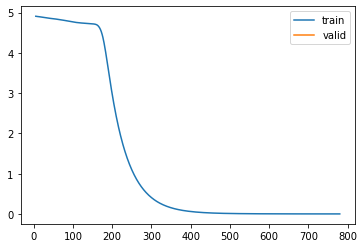The model fairly quickly learns the copy task, and reaches near 0 loss within a few hundred steps

#### Test inference and visualise attention

x = dls.train_ds.cuda()
x = x[None]
x[:,x.size(1)//2:], x[:,x.size(1)//2:].shape

(tensor([[  0,   1,  92, 105,  31,  84,  43, 112,  20,   7,  46,  90,  35,   5,
70, 110,  76,  19,   8,  60,  73,  42, 103,  81,  20,  13,  32,  49,
10,  39,  88, 122]], device='cuda:7'),
torch.Size([1, 32]))
learn.model.store_attention()
out = learn.model(x)

preds = out.argmax(-1)[:,x.size(1)//2:]
(preds[:,:-1]==x[:,x.size(1)//2+1:]).float().mean()

tensor(1., device='cuda:7')
attn = learn.model.get_attention_matrix()


We have 4 heads in our transformer, and 1 attention matrix per head:

The attention matrices shows us that mid way through the sequence the model starts paying attention to the first input sequence as expected. Each layers learns a bit different, but the combined output is good enough for perfect sequence copying. Note that there is no pattern to learn before this point, so the attention is just random noise. We also see that no token peeks ahead of it's own location, which we would expect from a language model with causal attention.

fig, axs = plt.subplots(1, 4, sharey=True, figsize=(16, 16))
for ax, mat in zip(axs, attn):
ax.matshow(mat)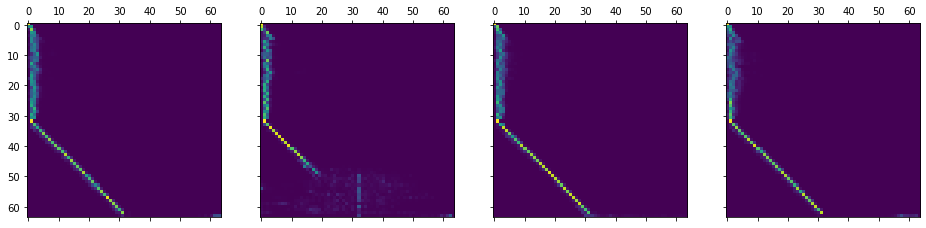del learn
torch.cuda.empty_cache()


### LSHLM

Note! Sequence length sl needs to be divisible by bucket_sizex2. So e.g. sl=64 -> bucket_size=32

n_hashes=4
bucket_size = 32
assert sl % (bucket_size * 2) == 0

model = LSHLM(vocab_sz=128, d_model=256, n_layers=1, n_heads=4, max_seq_len=sl,
bucket_size=bucket_size, n_hashes=n_hashes, causal=True)

learn = Learner(dls, model, loss_func=CrossEntropyLossFlat(ignore_index=-100),
learn.fit_one_cycle(n_epochs, 1e-3)

0 0.001956 0.000564 1.000000 01:26
learn.recorder.plot_loss()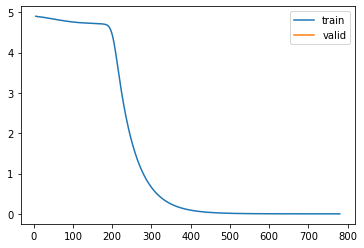del learn
torch.cuda.empty_cache()


### Test attention type switching

Let's see if we can train a model with full attention and evaluate with LSH-1.

model = LSHLM(vocab_sz=128, d_model=256, n_layers=1, n_heads=4, max_seq_len=sl,
bucket_size=bucket_size, n_hashes=n_hashes, causal=True,
use_lsh=False)  # disable LSH
learn = Learner(dls, model, loss_func=CrossEntropyLossFlat(ignore_index=-100),
learn.fit_one_cycle(n_epochs, 1e-3)

0 0.007624 0.000513 1.000000 00:19

Validation with full attention:

learn.model.use_lsh

False
%%time
learn.validate()

CPU times: user 1.4 s, sys: 848 ms, total: 2.25 s
Wall time: 2.34 s

(#2) [0.0005133371450938284,1.0]

Let's reset use_lsh to True

learn.model.use_lsh=True
learn.model.use_lsh

True
%%time
learn.validate()

CPU times: user 9.42 s, sys: 832 ms, total: 10.2 s
Wall time: 10.4 s

(#2) [4.350349426269531,0.154746875166893]

As expected validation with full attention performs better and faster

## Long sequence modelling

Increase the sequence length to 1024, similar what is used in the paper.

bs, sl = 32,1024
n_epochs = 4
train_sz = 50_000
valid_sz = 10_000
dls = DataLoaders.from_dsets(TwinSequence(sl, train_sz), TwinSequence(sl, valid_sz), bs=bs, shuffle=False, device='cuda')


Total training steps:

len(dls.train)*n_epochs

6248

### Transformer LM - full attention

model = TransformerLM(128, 256, d_ff=256, n_layers=1, n_heads=4, max_seq_len=sl, pos_enc='fixed',
attn_dropout=0, ff_dropout=0, emb_dropout=0)
learn = Learner(dls, model, loss_func=CrossEntropyLossFlat(ignore_index=-100),
learn.fit_one_cycle(n_epochs, 1e-3)

0 4.835779 4.836055 0.009745 01:48
1 0.000910 0.000835 0.999844 01:48
2 0.000025 0.000022 1.000000 01:48
3 0.000012 0.000012 1.000000 01:48
learn.recorder.plot_loss()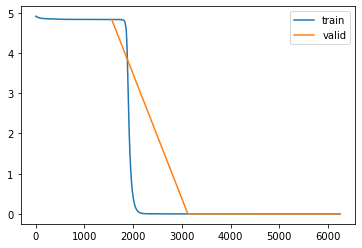del learn
torch.cuda.empty_cache()


The model learns the task perfectly, similar to the result reported in the paper.

### LSHLM

n_hashes=4
bucket_size = 64
assert sl % (bucket_size * 2) == 0

model = model = LSHLM(vocab_sz=128, d_model=256, n_layers=1, n_heads=4, max_seq_len=sl,
bucket_size=bucket_size, n_hashes=n_hashes, causal=True)
learn = Learner(dls, model, loss_func=CrossEntropyLossFlat(ignore_index=-100),
learn.fit_one_cycle(n_epochs, 1e-3)

0 4.835337 4.835193 0.009850 03:44
1 4.834929 4.834915 0.009803 03:48
2 4.834819 4.834768 0.009833 03:47
3 4.834756 4.834733 0.009887 03:47
learn.recorder.plot_loss()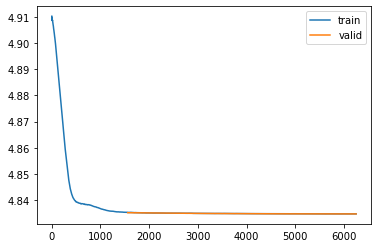del learn
torch.cuda.empty_cache()


The model struggles compared to full attention. The paper mentions 99.9% accuracy for this setup, but trained the model for 150k steps - much longer than in this case.

### LSHLM - single hashing round

We do a test similar to the one above, but just using a single hashing round in LSH.

n_hashes=1
bucket_size = 64
assert sl % (bucket_size * 2) == 0

model = LSHLM(vocab_sz=128, d_model=256, n_layers=1, n_heads=4, max_seq_len=sl,
bucket_size=bucket_size, n_hashes=n_hashes, causal=True)
learn = Learner(dls, model, loss_func=CrossEntropyLossFlat(ignore_index=-100),
learn.fit_one_cycle(n_epochs, 1e-3)

learn.recorder.plot_loss()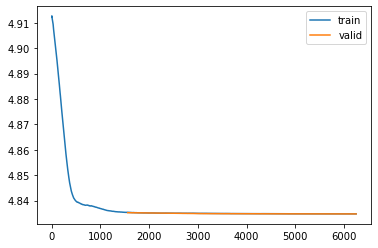del learn